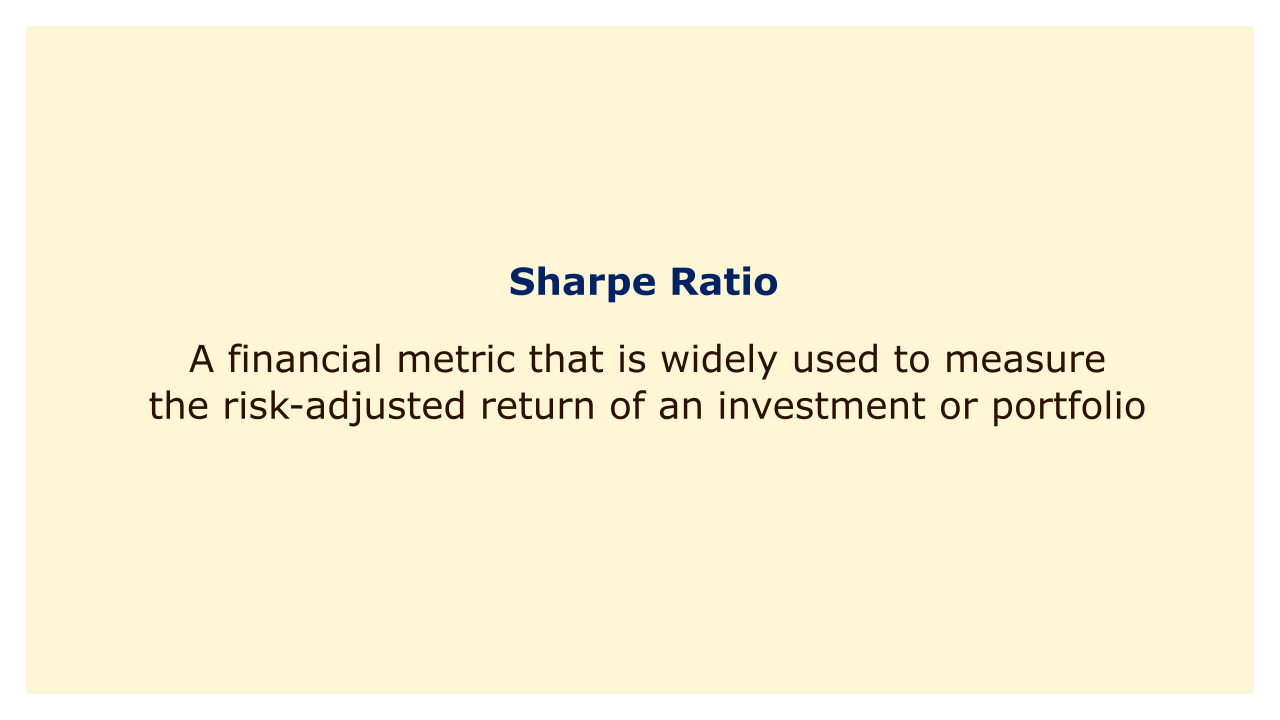# Sharpe RatioImage: Moneybestpal.com

### The Sharpe ratio is a financial metric that is widely used to measure the risk-adjusted return of an investment or portfolio. It was created by Nobel laureate William Sharpe in 1966 and is determined by dividing the excess return of the investment or portfolio over the risk-free rate by the excess return's standard deviation.

The Sharpe ratio calculates the additional return that an investment delivers for each unit of assumed risk. The Sharpe ratio indicates how well an investment fared relative to the level of risk it was exposed to. While a Sharpe ratio of 1 or greater is typically seen as a successful outcome, the optimal number may vary based on the particular investment plan and market circumstances.

For illustration, suppose an investment has a 10% yearly return, a 15% standard deviation, and a 3% risk-free rate. The investment's excess return is 7% (10% - 3%), and the excess return standard deviation is 15%. The investment produced 0.47 units of excess return for every unit of risk incurred, according to the Sharpe ratio, which would be computed as 0.47 (7%/15%).

The Sharpe ratio has developed into a crucial tool for investors as it facilitates comparing the performance of various assets with various levels of risk. It has, however, been criticism for a number of factors, including its reliance on the normal distribution of returns, sensitivity to outliers, and neglect of non-linear correlations between returns and risk. In spite of this, it is still a commonly used and accepted indicator in the investment world.
Tags